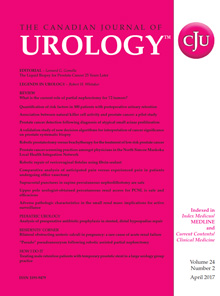# Details

Overestimation of prostate cancer mortality and other-cause mortality by the Kaplan-Meier method
Jun  2013 (Vol.  20, Issue  3, Pages( 6756 - 6760)
PMID: 23783043

## Text-Size +–

• ### INTRODUCTION:

To assess the extent of overestimation of the cumulative probability of death by the Kaplan-Meier method with the competing-risks regression analysis as reference approach.

### MATERIALS AND METHODS:

Data were derived from the screening arm of the Rotterdam branch of the European Randomized Study of Screening for Prostate Cancer (ERSPC). The screening arm consisted of 21210 men between the ages of 55 and 74 at study entry. Follow up concerning mortality was complete through 2008. Endpoints were 5 and 10 year cumulative probabilities of prostate cancer death and death from other causes. Relative bias was defined as the ratio of the cumulative probability of death as determined by the Kaplan-Meier method, relative to the cumulative probability obtained by the competing-risks analysis.

### RESULTS:

According to the Kaplan-Meier method, the 5 year cumulative probability of death from prostate cancer was 0.0101, compared with 0.0099 according to the competing-risk analysis [1.8% overestimation]. At 10 year, these numbers were 0.0347 and 0.0321, respectively [8.0% overestimation]. For death from other causes, the cumulative probabilities at 5 year were 0.0399 and 0.0397 according to the Kaplan-Meier and the competing-risks method [0.6% overestimation], respectively. At 10 year, the probabilities were 0.141 and 0.139 [1.7% overestimation], respectively.

### CONCLUSIONS:

When competing events are present, the competing-risks regression analysis is to be preferred over the Kaplan-Meier method in the estimation of the cumulative probability of the event of interest.

### Current Issue

October 2021, Vol.28 No.5